##Conic created as envelope of lines

Consider two points B, C on lines c, b correspondingly intersecting at A. Consider also a point D varying on a third line d. Let B', C' be the intersection points of DB, DC with lines c, b correspondingly. In the following statements tripoles/tripolars are taken with respect to triangle ABC.
 For D varying on d, lines B'C' envelope a conic (e), which is tangent to the sides a,b,c of triangle ABC.
 Line B'C' and the trilinear polar B*C* of D intersect at a point A* on line BC. Lines C'B* and B'C* intersect also on line BC at a point A2 which is the harmonic conjugate of A* with respect to B,C.
 The contact points of (e) with sides b, c are their intersection points with line d.
 The contact point of (e) with side a is the intersection point of side a with AS, where S is the tripole of d.
 The variable tangents B'C' have tripoles D' lying on the perspectrix of e.

Corollary-1 Every inscribed in a triangle ABC conic (e) is the envelope of the tripolars B'C' of points D' on its perspectrix.
Corollary-2 Let ABC be a triangle whose sides are tangent to a conic (e). Then, for every other triangle AB'C', whose sides are also tangent to the same conic and {A,B,C'}, {A,C,B'} are no-collinear, lines BC' and CB' intersect at a point D lying on the tripolar d = LS of point S. S is the intersection point of lines {AA1,BB1,CC1}, where A1 is the contact point of (e) with BC and C1, B1 are the intersection points of sides AB, AC correspondingly with the perspectrix of (e).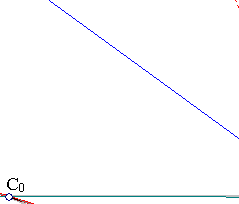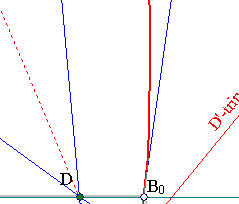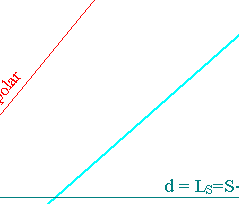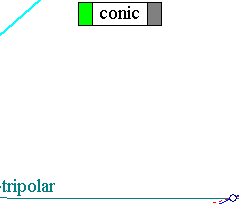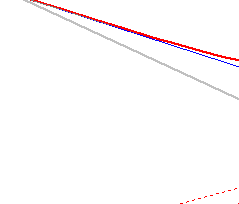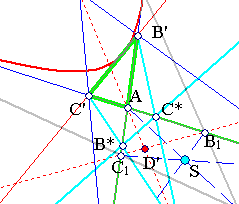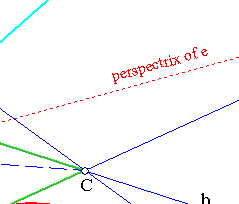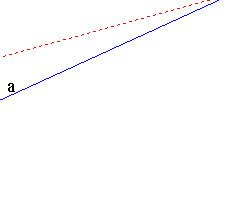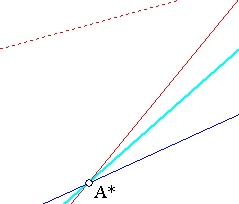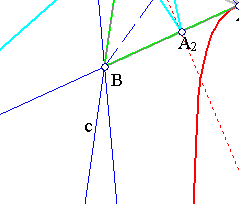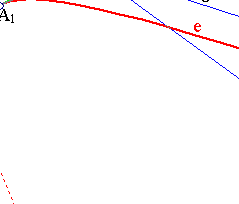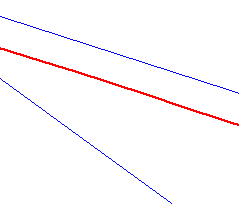These are generalizations of the analogous properties for hyperbolas studied in HyperbolaPropertyParallels.html . This general setting can be reduced to the aforementioned one by a projective transformation F fixing lines a, b, c and sending line d to infinity. Then the envelope of C'B' is mapped to an hyperbola, hence the stated result. The result on the tangency is related to the fact that B0, C0 are precisely the points send by F to infinity. The proof of the corollaries follows also by corresponding corollaries valid for the transformed (under F) conic.

For some complementary remarks on the various incidence relations of the figure see InscribedConicAsEnvelope.html .